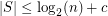# subset sum

## Sets with distinct subset sums ★★★

Author(s): Erdos

Say that a sethas distinct subset sums if distinct subsets ofhave distinct sums.

Conjecture   There exists a fixed constantso thatwheneverhas distinct subset sums.

Keywords: subset sum